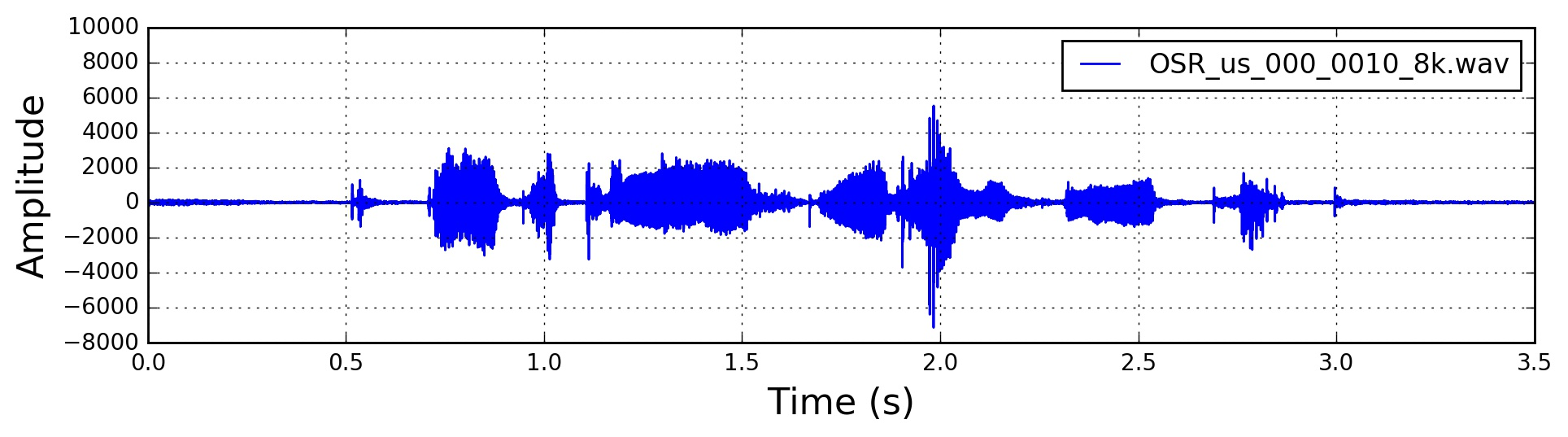# negative value of signalscan we say there is no sound produced when the amplitude is 0? if yes what can we say when the amplitude is negative?

• Short answer: it means your signal is either zero or negative. If you really want a meaningful answer, you should add more information about the source/ nature of your signal and why you have troubles interpreting the observed behavior – Irreducible May 3 at 7:19

## 2 Answers

I believe you are talking about soundwaves only, so I'll approach the answer from there.

The instant values a signal takes don't tell much about the sound it may generate. If a signal is zero at a certain instant $$t_0$$, it doesn't mean that at that moment there is silence. A single sample can't represent any sound because only oscillations can generate sound. I hope you see why a single sample can't possibly represent an oscillation of any kind.

Take for example a perfect sinewave. The signal $$x(t)=\sin(t)$$ is $$0$$ for infinite values of $$t$$, but the sound you hear is nonetheless uniform and constant. Also, it takes both positive and negative values, but the note you hear is always the same.

If a signal is $$0$$ for a given period of time though, then for that interval there will be silence indeed. In fact, if a signal is constant for a given period of time (not necessarily $$0$$), you will hear no sound at all (but you would be wasting power nonsensically).

In a nutshell, the instant values of a signal represent no sound whatsoever. It's the change over time of these values that can represent and generate sounds.

• what if our signal is negative for a given period of time – ar4n m3t May 4 at 5:21
• @ar4nm3t As long as it is varying, there will be sound. Whether it is negative or positive doesn't matter. – Tendero May 5 at 15:20

Truthfully, the zero isn't really zero at all, just a relative value.

When an audio signal is recorded, the signal values represent variations in air pressure around a central average value.

When an audio signal is played back, the zero represents the resting position of the speaker cone.

When a signal as no ampitude (all samples are zero) then there is no sound. An individual sample (as already pointed out) is meaningless in this context. However, a DC signal (all samples the same value) will not make a sound either. (With coupling capacitors, the speaker cone will return to resting position, but that is a different story).

Ced